Courses

# Lane Changing Models Civil Engineering (CE) Notes | EduRev

## Civil Engineering (CE): Lane Changing Models Civil Engineering (CE) Notes | EduRev

The document Lane Changing Models Civil Engineering (CE) Notes | EduRev is a part of the Civil Engineering (CE) Course Transportation Engineering.
All you need of Civil Engineering (CE) at this link: Civil Engineering (CE)

Basic Lane changing model

The basic lane change model is described using the framework shown in Figure 15:1. The subject vehicle in the current lane tries to change direction either to its left or to its right. If the gap in the selected lane is acceptable the lane change occurs or else it will remain in the current lane

Classification of Lane change

The classification of lane changes is done based on the execution of the lane change and accordingly two type of lane changes exists.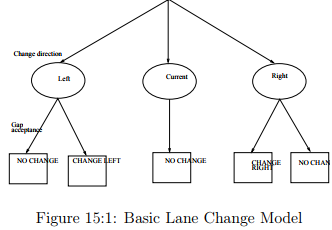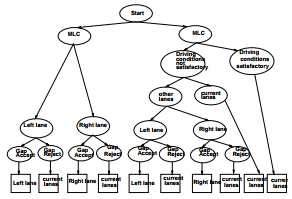Figure 15:2: Discretionary Lane change model

Mandatory Lane Change (MLC):

Mandatory lane change (MLC) occurs when a driver must change lane to follow a specified path Suppose if a driver wants to make a right turn at the next intersection the he changes to the right most lane which is referred as Mandatory Lane change.

Discretionary Lane Change (DLC):

Discretionary lane change (DLC) occurs when a driver changes to a lane perceived to offer better traffic conditions, he attempts to achieve desired speed, avoid following trucks, avoid merging traffic, etc. Suppose if a driver perceives better driving conditions in the adjacent lane then he makes a Discretionary Lane change.

MLC and DLC Model

The lane changing model structure is shown in Figure 15:2. The MLC branch in the top level corresponds to the case when a driver decides to respond to the MLC condition. Explanatory variables that affect such decision include remaining distance to the point at which lane change must be completed, the number of lanes to cross to reach a lane connected to the next link, delay (time elapsed since the MLC conditions apply), and whether the subject vehicle is a heavy vehicle (bus, truck, etc..,). Drivers are likely to respond to the MLC situations earlier if it involves crossing several lanes. A longer delay makes a driver more anxious and increases the likelihood of responding to the MLC situations. And finally, due to lower maneuverability and larger gap length requirement of heavy vehicles as compared to their non heavy counterparts, they have a higher likelihood of responding to the MLC conditions.

Decision making

The MLC branch corresponds to the case where either a driver does not respond to an MLC condition, or that MLC conditions do not apply. A driver then decides whether to perform a discretionary lane change (DLC). This comprises of two decisions: whether the driving conditions are satisfactory, and if not satisfactory, whether any other lane is better than the current lane. The term driving conditions satisfactory implies that the driver is satisfied with the driving conditions of the current lane. Important factors affecting the decision whether the driving conditions are satisfactory include the speed of the driver compared to its desired speed, presence of heavy vehicles in front and behind the subject, if an adjacent on ramp merges with the current lane, whether the subject is tailgated etc.

If the driving conditions are not satisfactory, the driver compares the driving conditions of the current lane with those of the adjacent lanes. Important factors affecting this decision include the difference between the speed of traffic in different lanes and the driver’s desired speed, the density of traffic in different lanes, the relative speed with respect to the lag vehicle in the target lane, the presence of heavy vehicles in different lanes ahead of the subject etc. In addition, when a driver considers DLC although a mandatory lane change is required but the driver is not responding to the MLC conditions, changing lanes opposite to the direction as required by the MLC conditions may be less desirable.

If a driver decides not to perform a discretionary lane change (i.e., either the driving conditions are satisfactory, or, although the driving conditions are not satisfactory, the current is the lane with the best driving conditions) the driver continues in the current lane. Otherwise, the driver selects a lane from the available alternatives and assesses the adjacent gap in the target lane.. When trying to perform a DLC, factors that affect drivers’ gap acceptance behavior include the gap length, speed of the subject, speed of the vehicles ahead of and behind the subject in the target lane, and the type of the subject vehicle (heavy vehicle or not). For instance, a larger gap is required for merging at a higher travel speed. A heavy vehicle would require a larger gap length compared to a car due to lower maneuverability and the length of

Lane changing models

Most models classify lane changes as either mandatory or discretionary lane change. This separation implies that there are no trade-offs between mandatory and discretionary considerations. For example, a vehicle on a freeway that intends to take on of-ramp will not overtake a slower vehicle if the distance to the off-ramp is below a threshold, regardless of the speed of that vehicle. Furthermore, in order to implement MLC and DLC model separately, rules that dictate when drivers begin to respond to MLC conditions need to be defined. However, this point is unobservable, and so only judgment-based heuristic rules, which often are defined by the distance from the point where the MLC must be completed, are used. Just like the judgement based lane changing models ,there also exist lot of other models like general acceleration based lane changing models and gap acceptance based lane changing models

Forced merging model

If the gap on the target lane is not acceptable then the subject vehicle forces the lag vehicle on the target to decelerate until the gap is acceptable. This process is known as Forced merging. At every discrete point in time, a driver is assumed to (a) evaluate the traffic environment in the target lane to decide whether the driver intends to merge in front of the lag vehicle in the target lane and (b) try to communicate with the lag vehicle to understand whether the driver’s right of way is established. If a driver intends to merge in front of the lag vehicle and right of way is established, the decision process ends and the driver gradually move into the target lane. We characterize this instant by state M, where M denotes start forced merging. This process may last from less than a second to a few seconds. If right of way is not established, the subject continues the evaluation/communication process (i.e., remains in state M) during the next time instant.

Cooperative Merging

The models discussed so far assume that lane changing is executed through gap acceptance. However, in congested traffic conditions acceptable gaps may not be available, and so other mechanisms for lane changing are needed. For example, drivers may change lanes through courtesy and cooperation of the lag vehicles on the target lane that will slow down in order to accommodate the lane change. In other cases, some drivers may become impatient and decide to force in to the target lane and compel the lag vehicle to slow down.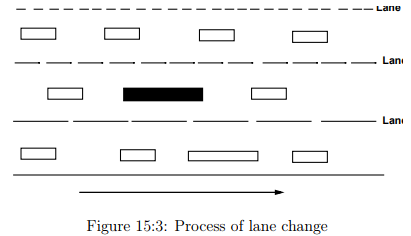Discretionary lane changing process

The discretionary lane changing process is modeled as a sequence of the following three steps:
1. Decision to consider a lane change,
2. Check for the feasibility,
3. Gap acceptance
Lane changing process is explained using the example which is shown in Figure 15:3. The subject vehicle in lane 2 makes a decision to consider a lane change and then it selects a target lane which may be either lane 1 or lane 3. Then it checks for the feasibility of lane change. Now this subject vehicle accepts the gap in the target lane to make a lane change.

Desire to change the lane

Decision to change the lane in discretionary lane change conditions may be taken due to a number of factors but basically what the driver has in mind should be higher utility in the target lane which may be for example higher speed. Here we use the equation suggested by Gipps (1986) to find if it is possible for the driver to attain his desired speed within the existing space difference between his vehicle and the preceding vehicle in the current lane. If required space difference is not available, the driver is assumed to decide lane change. The relation is given as: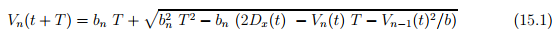where, Vn(t + T) is the maximum safe speed for vehicle n with respect to the preceding vehicle at time (t+T), Vn(t) is the velocity nth vehicle, Vn−1(t) is the velocity n-1th vehicle, bn (< 0) is the most severe braking the driver is prepared to undertake, T is the time between consecutive calculations of speed and position, b is an estimate of bn−1 employed by the driver of vehicle n, and Dx(t) is the distance between front of subject vehicle and rear of leading vehicle at t. The driver is assumed to decide to change lane if Dx is more than the existing space gap between the subject vehicle and preceding vehicle in current lane.

Check for feasibility

The lane change is said to be feasible if the chance that the subject vehicle would collide at the rear of preceding vehicle in the target lane and the chance that the lag vehicle in the target lane would collide at the rear of the subject vehicle is avoided. To check if the subject vehicle would collide at the rear of preceding vehicle in the target lane we consider the subject vehicle as n and preceding vehicle in the target lane as n-1.Then we substitute the values in the equation 15.1. If the maximum safe speed can be attained in the time T with a deceleration less than the maximum possible deceleration of the vehicle we say that the lane change is feasible.

To check if the lag vehicle after sighting the subject vehicle in the target lane, would collide at the rear of subject vehicle in the target lane. For this we consider the lag vehicle as N and subject vehicle in the target lane as n-1. Also we have to consider that the space difference available will be changed as both the vehicles would have moved a distance during the lane change. Then we substitute the values in the equation 15.1. If the maximum safe speed can be attained in the time T by the lag vehicle with a deceleration less than the maximum possible deceleration of the vehicle we say that the lane change is feasible

Gap acceptance

A gap is defined as the gap in between the lead and lag vehicles in the target lane (see Figure 15:4). For merging into an adjacent lane, a gap is acceptable only when both lead and lag gap are acceptable. Drivers are assumed to have minimum acceptable lead and lag gap lengths which are termed as the lead and lag critical gaps respectively. These critical gaps vary not only among different individuals, but also for a given individual under different traffic conditions. Most models also make a distinction between the lead gap and the lag gap and require that both are acceptable. The lead gap is the gap between the subject vehicle and the vehicle ahead of it in the lane it is changing to. The lag gap is defined in the same way relative to the vehicle behind in that lane. The critical gap lengths are assumed to be log normally distributed The critical gap for driver n at time t is assumed to have the following relation.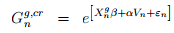where,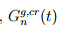is the critical gap measure for gap G perceived by driver n at time step t,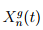is the explanatory variable used to characterize mean, εn is the random term follows log normal distribution, and αis the parameter of driver specific random term vn. Assuming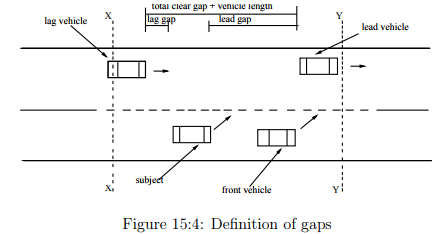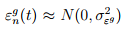the conditional probability of acceptance of a gap p(g) considering that the probability of lead gap acceptance (p(lead)) and lag gap (p(lag)) acceptance as two independent events is probability that the lead and lag gaps are accepted. That is: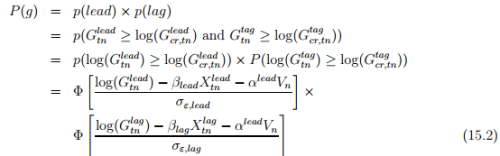where (X) means X follows standard normal distribution ,N(0,1)

Numerical Example

For the given state of traffic predict if the subject vehicle in the figure 5 would initiate a lane change.if yes what is the feasibility and probability of lane change. Given is the midblock section of 2 lane highway with no other blocks in either of the lane. Neglect lateral

acceleration. Consider update time 1 sec. Maximum deceleration driver ready to apply is -2 m/s2 and maximum acceleration feasible is -2.2 m/s2 Assume that lane change take 1 second. Given: σ lead =2, σlead = 3 ,Glead =40m, Glag =50m ,β lead = β lag = 1 ,Xnllead = Xnlag = 0.8, V lead n = V lag n = 0.7 , α leadlag = 1.2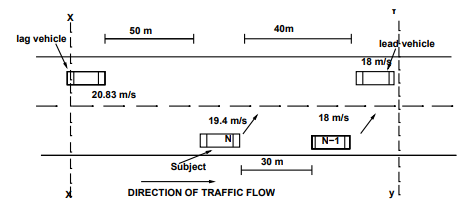Solution Step 1. Decision to change the lane: In the case of discretionary lane change, the decision to change the lane is taken by the driver when he finds higher utility in any other lane. Here, we consider higher speed or desired speed as higher utility. Let the desired speed be 25 m/s2 . Considering the subject vehicle as vehicle n and the vehicle preceding it in the current lane as vehicle n-1, we calculate the minimum distance required by the subject vehicle to attain the desired speed in a time T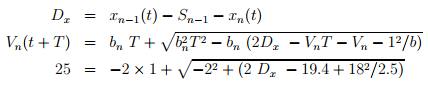The Dx in this problem is 155 m, which means that the subject vehicle requires at least 155 m to reach his desired speed. But the gap available is 30 m. So decision is to change the lane or trigger DLC.

Step 2. Check for the feasibility of lane change: A lane change is said to be feasible if the subject vehicle is able to maintain maximum safe speed with respect to the preceding vehicle in the target line. In order to find the maximum safe speed possible for the subject vehicle to avoid collision we consider the subject vehicle as N and preceding vehicle in the target lane as N-1. Then we substitute the values in the second equation. Vn(t + T) = −2 × 1 + [−2 2 + 22(40) − 19.4 + 182/2.5]1/2 = 17.6m/s And the deceleration required = (17.6- 19.4)/1 = -1.79 m/s2 Since -1.79 m/s2 less than -2.2 m/s2 the lane change feasible to avoid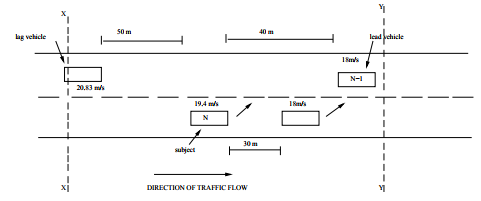collision with the lead vehicle in the target lane. Now we have to check if the lag vehicle in the target line would be able to avoid the collision with the subject vehicle after the lane change. For this we take lag vehicle as Nth vehicle and subject vehicle as N − 1th vehicle Here,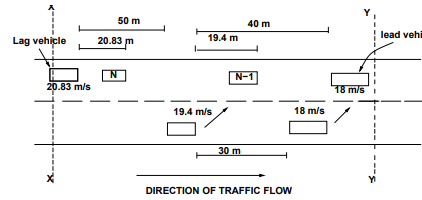Dx = 50 + 19.4−20.83 = 48.5m as the lag vehicle and subject vehicle would have moved some distance during the lane change duration of 1 second. These distances are 20.83 m and 19.4 m respectively. Vn(t + T) = −2x1 + [−22 + 22(48.5) − 20.83 + 19.42/2.5]1/2 = 19.38m/s The deceleration required to be applied by the lag vehicle in the target lane to avoid collision with the subject vehicle = (19.38 − 20.83)/1 = −1.44m/s2 . Since -1.44 m/s2 ¡ -2.2 m/s2 the lane change feasible to avoid collision of the lag vehicle in the target lane.

Step 3. Check for the gap acceptance of lane change in the given state of traffic: Here we find that the lag gap that was available is 50 m and the lead gap is 40 m.using the equation 2 we get,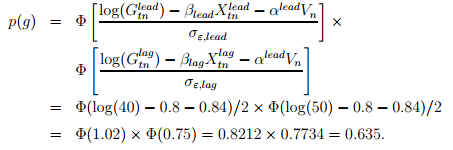This means that a given driver would opt for a lane change in the the given condition with a probability of 0.635.

Average Delay for Lane change

The blockage length and the average delay for the lane change are calculated based on the following formulae.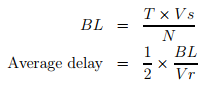where, T = Total time of headways rejected, BL = blockage length, Vs = stream velocity, Vr = relative velocity, N = number of acceptable gap

Numerical Example

In a two lane, one way stream of 1000 vph with 360 vehicles in Lane A and the remaining vehicles in lane B. 8% of the vehicles in lane A have gaps less than 1 sec and 18% of the vehicles in lane A have gaps less than 2 sec. Compute the time during which vehicles in Lane B may not change to Lane A in 1 hour. Assume driver requires one second ahead and behind in making a lane change.

Solution Total acceptable time for lane change in an hour = 3600 - total rejected headway - total clearance time. Given that 0 to 1 second Gaps is 8% of 360 = 29 and 1 to 2 second Gaps is (18-8) % of 360 = 36. Total = 65 Gaps. Time spent in Gaps 0 to 1 second = 29 x .5 = 14.5 sec, and Time spent in Gaps 1 to 2 second = 36 x 1.5 = 54.0 sec. As 65 Gaps are rejected, Acceptable Gaps are (360-65) = 295 Gaps. In this 295 Gaps clearance time = 295 x 2 = 590 sec. Time lost in rejected gap = 14.5+54=68.5. Therefore, Total time left in one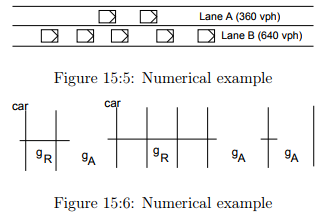hour to accept Gap=(3600 - 590 - 68.5) = 2941 sec. Vehicle can change lane in (2941/3600) = 81.7% of the Total time. Vehicle is prevented from changing lane in 18.3% of the time.

Numerical Example

In a two lane, one way stream of 1000 vph with 360 vehicles in Lane A and the remaining vehicles in lane B. 8% of the vehicles in lane A have gaps less than 1 sec and 18% of the vehicles in lane A have gaps less than 2 sec. Compute the average waiting for the driver to make a lane change. Assume driver velocity in lane B = 40 kmph and stream velocity = 50 kmph. Solution: The average length of headways and portions of head ways of insufficient length for a lane change, which may be considered as general blockages moving in the stream. Division of this Blockage length by the relative speed determines potential total delay time of the blockade. Finally since a delayed vehicle is as likely to be at the head as at the tail of such a blockade at the moment of desired lane change, the total delay must be divided by 2 for average delay time.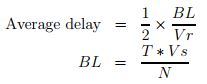where T = 3600 × 18.3% = 658.8, Vs = 50 kmph, N = acceptable gaps. So 0 to 2 second Gaps = 18 % of 360 = 65. Therefore N = 360-65 = 295. BL =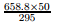= 111.6. In the above figure the vertical lines are the center line of the cars and gr, ga represents the acceptable and rejected gaps respectively.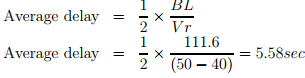The document Lane Changing Models Civil Engineering (CE) Notes | EduRev is a part of the Civil Engineering (CE) Course Transportation Engineering.
All you need of Civil Engineering (CE) at this link: Civil Engineering (CE)Use Code STAYHOME200 and get INR 200 additional OFF Use Coupon Code
All Tests, Videos & Notes of Civil Engineering (CE): Civil Engineering (CE)

### Top Courses for Civil Engineering (CE)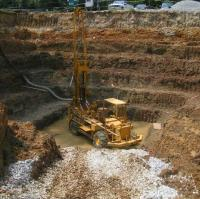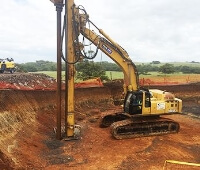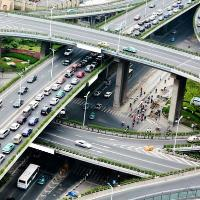## Transportation Engineering

23 videos|66 docs|39 tests

### Top Courses for Civil Engineering (CE)Track your progress, build streaks, highlight & save important lessons and more!

,

,

,

,

,

,

,

,

,

,

,

,

,

,

,

,

,

,

,

,

,

;